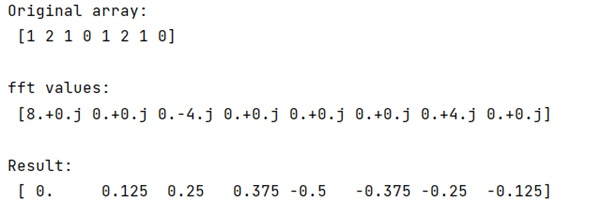# How to extract frequency associated with fft values?

Learn, how to extract frequency associated with fft values in Python?
Submitted by Pranit Sharma, on February 17, 2023

NumPy is an abbreviated form of Numerical Python. It is used for different types of scientific operations in python. Numpy is a vast library in python which is used for almost every kind of scientific or mathematical operation. It is itself an array which is a collection of various methods and functions for processing the arrays.

## Extracting frequency associated with fft values

The numpy.fft.fft() computes the one-dimensional discrete Fourier Transform. This function computes the one-dimensional n-point discrete Fourier Transform (DFT) with the efficient Fast Fourier Transform (FFT) algorithm [CT].

We will use numpy.fft.fftfreq() which tells us the frequencies associated with the coefficients.

Let us understand with the help of an example,

## Python code to extract frequency associated with fft values

```# Import numpy
import numpy as np

# Creating a numpy array
arr = np.array([1,2,1,0,1,2,1,0])

# Display original array
print("Original array:\n",arr,"\n")

# Computing fft values
res = np.fft.fft(arr)

# Display fft values
print("fft values:\n",res,"\n")

# Calculating frequencies
fre = np.fft.fftfreq(len(arr))

# Display Result
print("Result:\n",fre)
```

Output: